# RFEM – Online Manual

Online manuals, introductory examples, tutorials, and other documentation.

# 11.6.4 Formulas in Tables and Dialog Boxes

### Formulas in Tables and Dialog Boxes

The equations stored in the formula editor can be used both in the table cells and in the text boxes of dialog boxes. Since tables and dialog boxes are interactive, you can access the formulas in both input modes.

Formulas in tables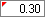When cells are marked by a yellow or red flag (triangle) in the upper left corner, a formula has been linked (see Figure 11.134). Click the flag to open the formula editor.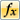To link a "normal" cell with a formula, place the pointer in this cell and open the formula editor by using the button shown on the left.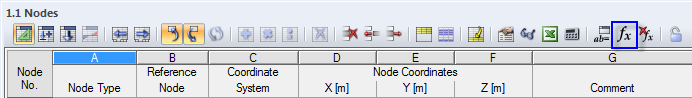Figure 11.138 Edit Formulas button in the table toolbar

A red flag means that there is an error in the formula. This corresponds to the red formula line in the formula editor. The formula should be corrected.

Formulas in dialog boxes

The parametrized input has been developed primarily for the application in tables. However, it is also possible to use formulas in dialog boxes.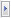A function button to the right of the text boxes in dialog boxes indicates that they can be linked with formulas.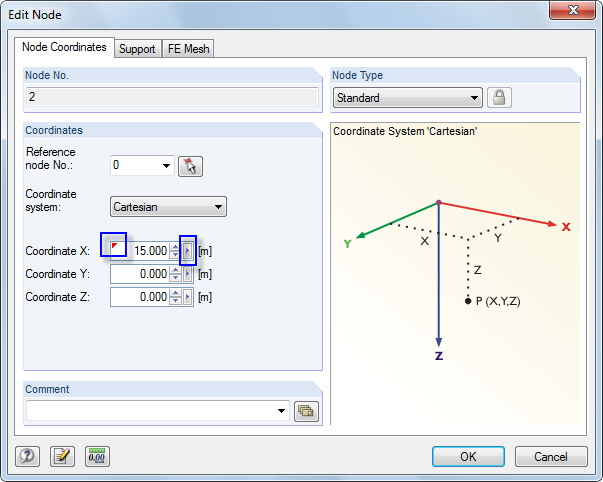Figure 11.139 Dialog box with linked formula and function button

If the text box has already been linked with a formula, it is marked by a yellow flag (or red flag in case of incorrect input) like a cell.

Click thefunction button to open the shortcut menu shown in Figure 11.135. You can access the formula editor in this menu.